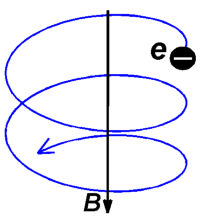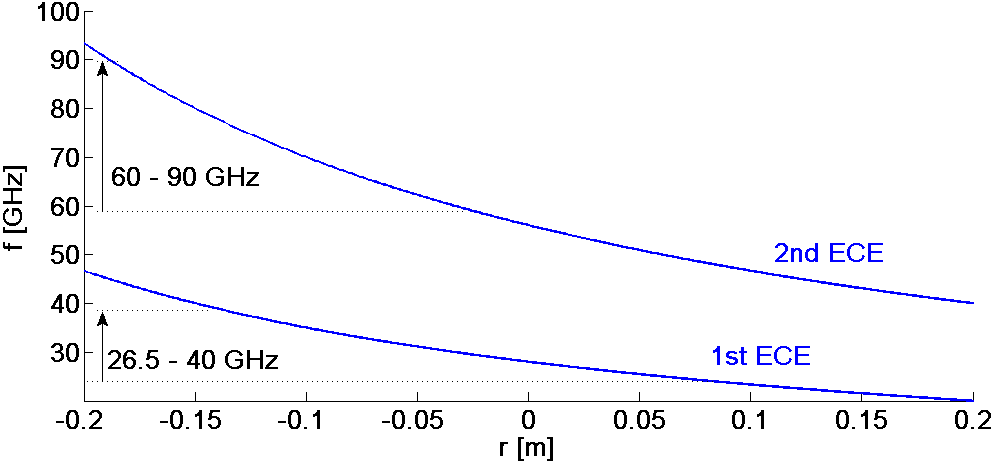The Academy of Sciences of the Czech rebublic - ASCR

 Measured quantities: Radial profile of electron temperature Spatial resolution: ~ 1 cm Temporal resolution: 1 µs Responsible person: J. Zajac

Diagnostic description:Fig. 1: Gyromotion of the electron
along the magnetic field line

Radiometer is a sensitive microwave receiver which measures the temperature of an object by measurement of the power level of the thermal noise emitted by the object. Radiometers are widely used in radioastronomy, but also as the diagnostic of hot magnetized plasma.

Plasma radiates in a wide electromagnetic spectrum range. The waves which are well defined, can be used for a specific diagnostic purpose. The electron cyclotron emission (ECE) is a radiation generated by a gyration of the electron along the magnetic field line (Fig. 1). The frequency of the cyclotron emission is then given by:where n is an integer of the harmonics, B is the magnetic field strength, e and me are the electron charge and mass, respectively. The toroidal magnetic field B(r), and thereby the freqency fe of ECE, is inversely proportional to radius r. That means that the inner electrons emit higher frequency then the outer electrons. The specification of tokamak plasma is, that usually the second harmonic frequency of ECE radiates as so called "black body", where the power of the emission is directly proportional to the electron temperature. Therefore, the electron temperature can be measured by the calibrated radiometer, where fe gives us the radial position of the radiating electrons due to known dependence of  B(r) – see Fig. 2 below.

The present radiometer on the COMPASS tokamak measures in two frequency bands 26.5 – 40 GHz and 60 – 90 GHz. It is a double superheterodyne, the microwave band is downconverted and divided into frequency 16 channels.

The band 60 – 90 GHz is devoted to the measurement of the electron temperature on the 2nd harmonics ECE and 26.5 – 40 GHz band is used for the study of the electron Bernstein waves (EBW) on the 1st harmonics.Fig. 2.: Dependance of fe on the radius in the COMPASS tokamak for the central magnetic field BT = 1 T. The frequency of radiometer bands are also shown